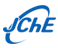SCI和EI收录∣中国化工学会会刊

›› 2017, Vol. 25 ›› Issue (9): 1273-1281.

• Chemical Engineering Thermodynamics •

### A new model to predict the densities of nanofluids using statistical mechanics and artificial intelligent plus principal component analysis

F. Yousefi, Z. Amoozandeh

1. Department of Chemistry, Yasouj University, Yasouj, 75914-353, Iran
• Received:2016-02-17 Revised:2016-10-24 Online:2017-10-11 Published:2017-09-28

### A new model to predict the densities of nanofluids using statistical mechanics and artificial intelligent plus principal component analysis

F. Yousefi, Z. Amoozandeh

1. Department of Chemistry, Yasouj University, Yasouj, 75914-353, Iran
• 通讯作者: F. Yousefi,E-mail:fyousefi@mail.yu.ac.ir

Abstract: In this work, some thermodynamic properties of nanofluids such as Sb2O5; SnO2/(EG + H2O), ZnO/(EG + H2O), Al2O3/(EG + H2O), ZnO/(PEG + H2O), ZnO/PEG, and TiO2/EG were estimated from the extended Tao-Mason equation of state, together with the Pak and Cho equation in various temperature, pressure, and volume fractions. The equations of state using minimum input data and density at room temperature as scaling constants, were developed to estimated densities of the above mentioned nanofluids. Furthermore, the artificial neural network plus principal component analysis (PCA) technique (with 20 neuron in hidden layer) was performed over the whole range of available conditions. The AADs of the calculated molar densities of all nanofluids using the EOS and ANN at various temperatures and volume fractions are 1.11% and 0.48%, respectively. In addition, the heat capacity and isentropic compressibility of the above mentioned nanofluids are predicted using obtained densities of EOS with the Pak and Cho equation.

Key words: Nanofluids, ANN, Equation of state, Model